CBSE Class 12 Sample Paper for 2020 Boards

Class 12
Solutions of Sample Papers and Past Year Papers - for Class 12 Boards

## y + 2z = 7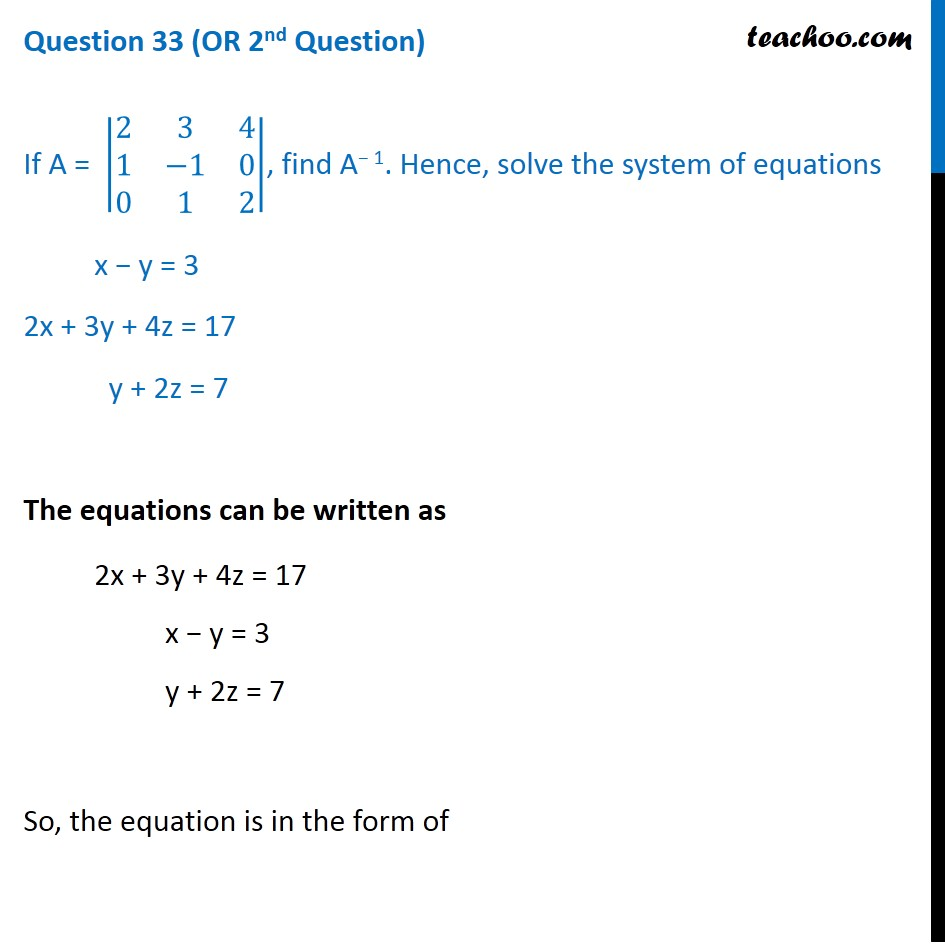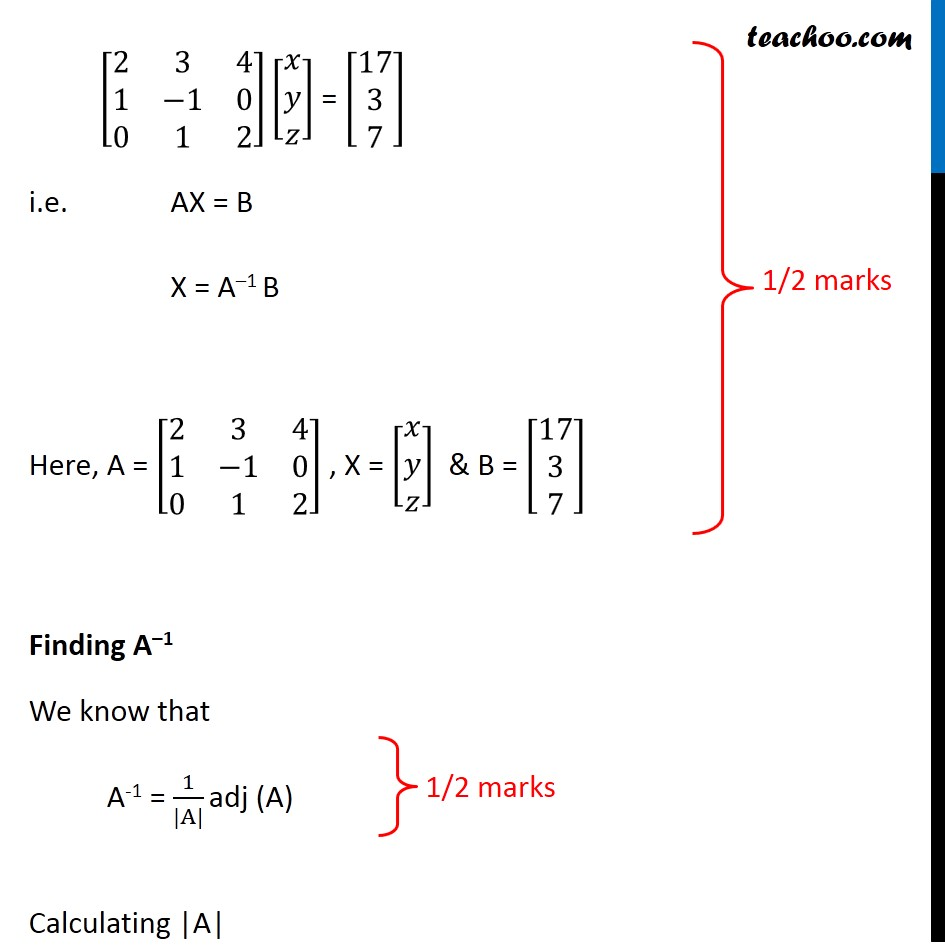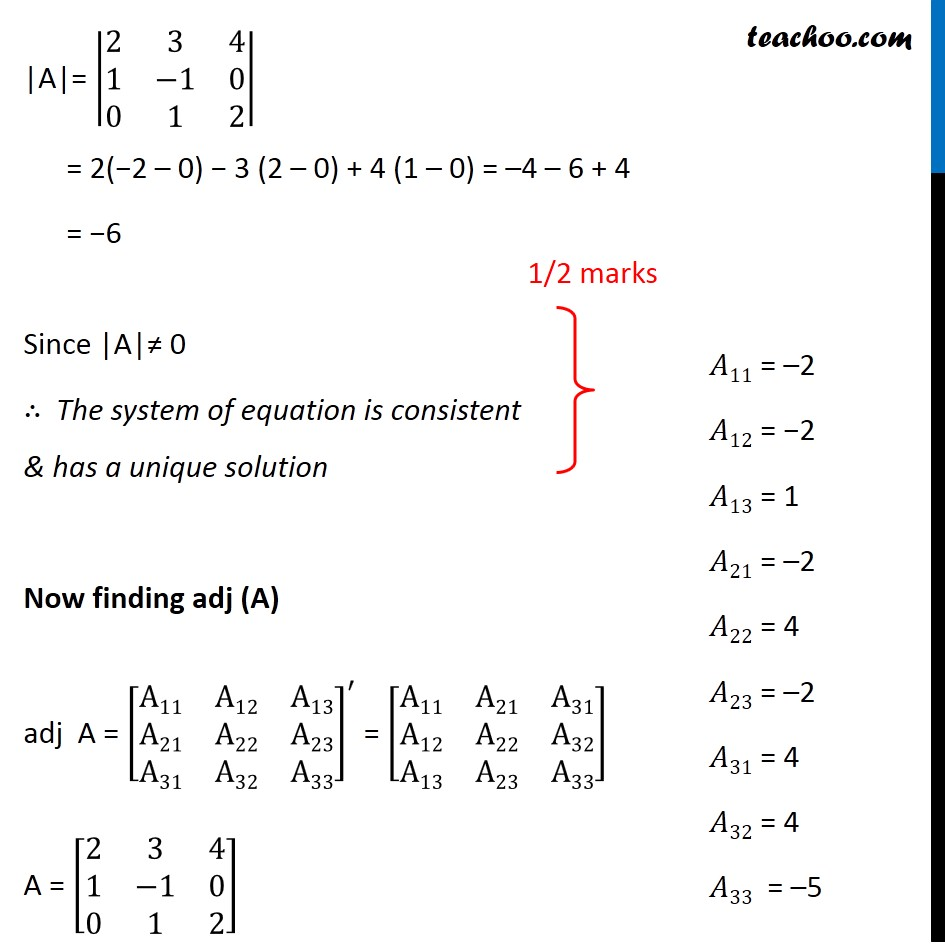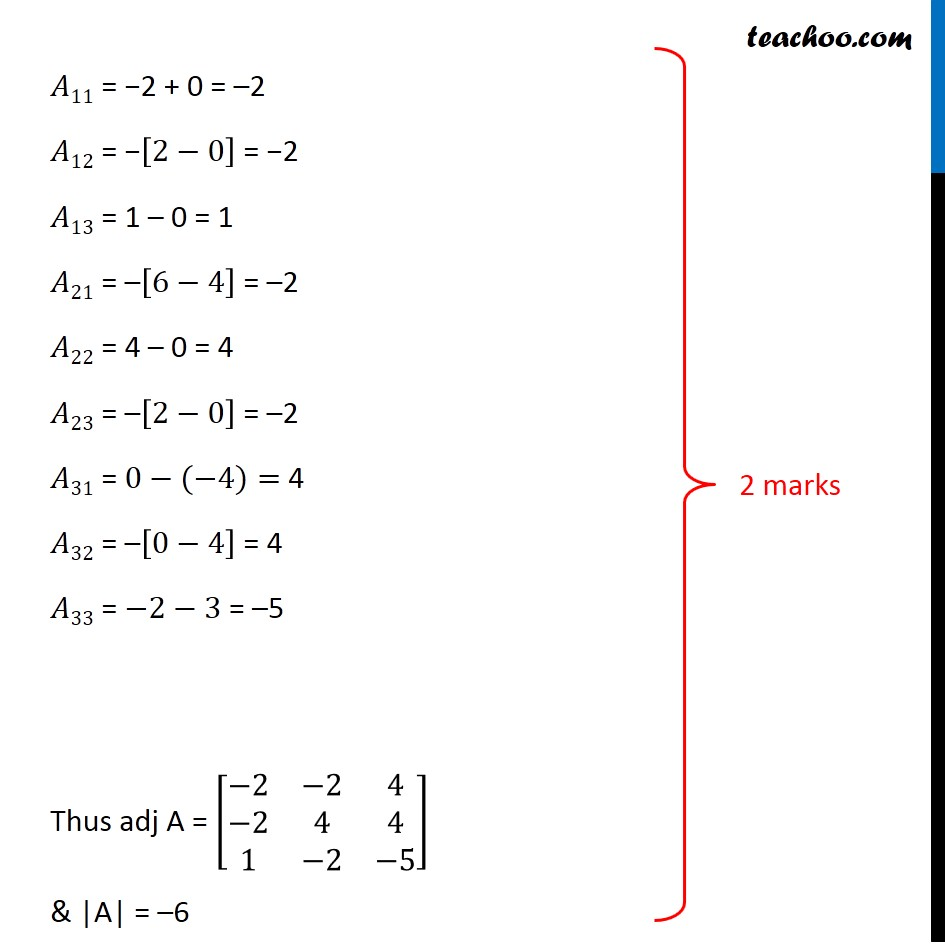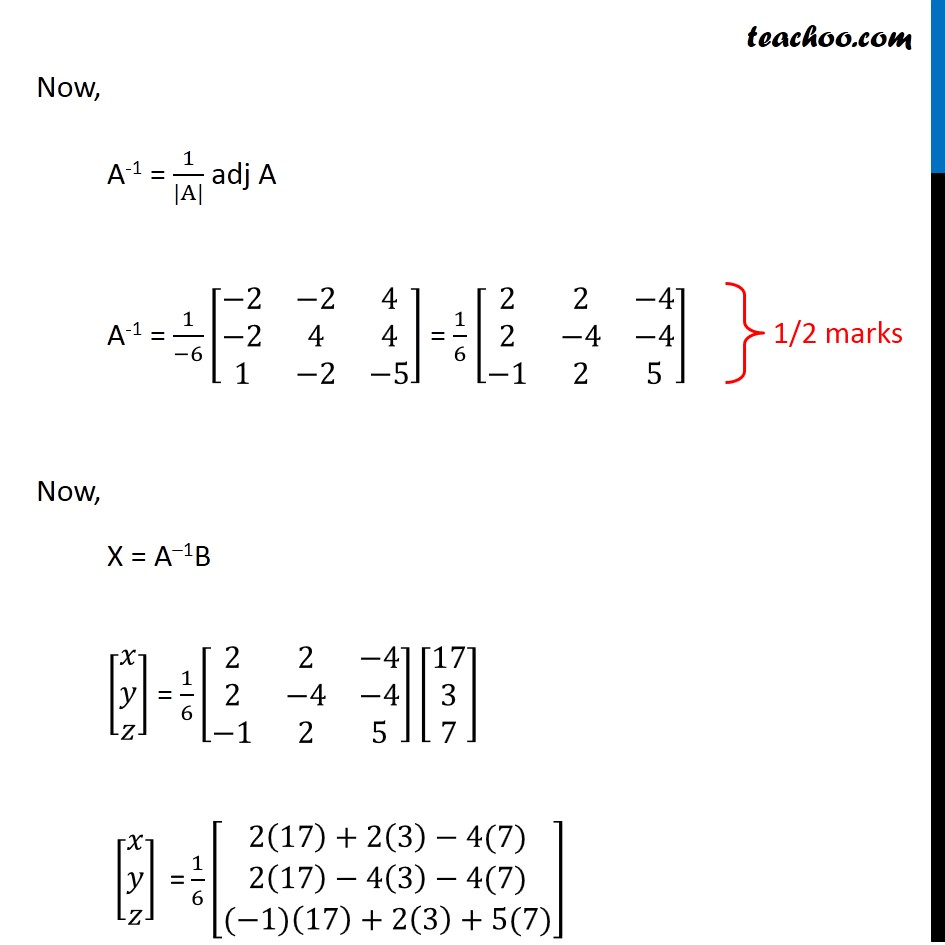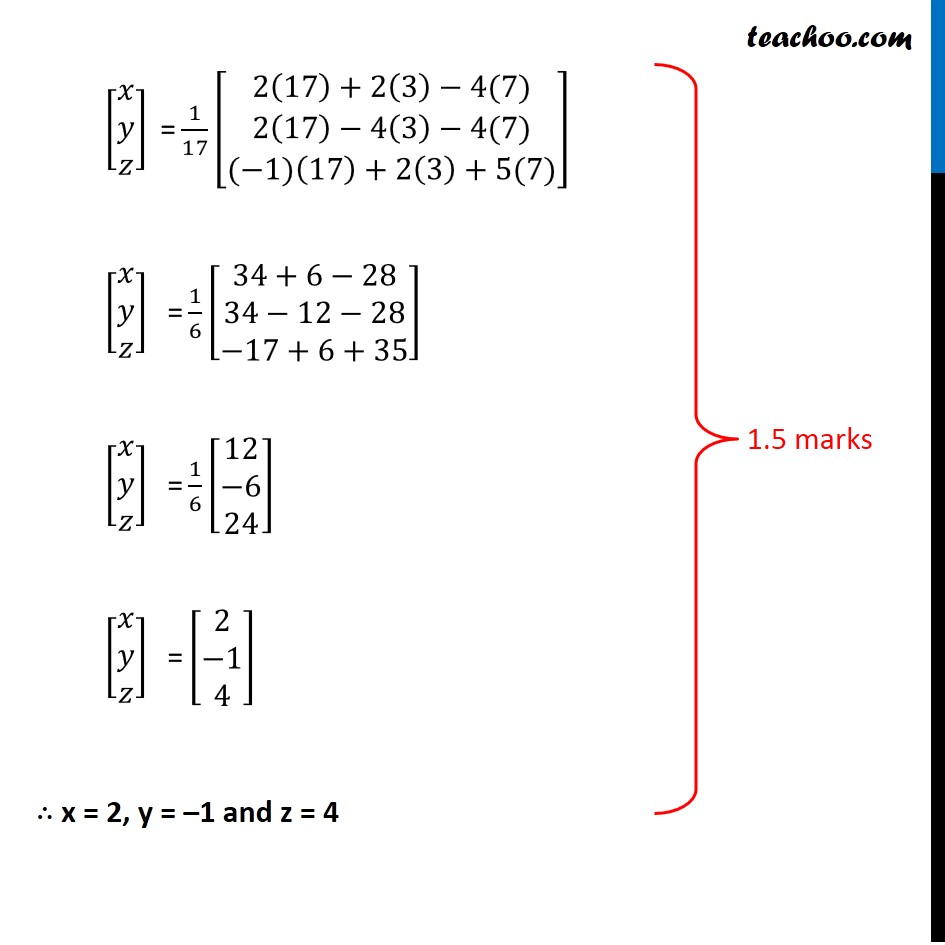Get live Maths 1-on-1 Classs - Class 6 to 12

### Transcript

Question 33 (OR 2nd Question) If A = |2 3 4 1 -1 0 0 1 2|, find A− 1. Hence, solve the system of equations x − y = 3 2x + 3y + 4z = 17 y + 2z = 7 The equations can be written as 2x + 3y + 4z = 17 x − y = 3 y + 2z = 7 So, the equation is in the form of [2 3 4 1 -1 0 0 1 2] [ x y z] [17 3 7] i.e. AX = B X = A–1 B Here, A = [ 2 3 4 1 -1 0 0 1 2], x = [x y z] & B = [17 3 7] Finding A–1 We know that A-1 = 1/(|A|) adj (A) Calculating |A| |A|= [ 2 3 4 1 -1 0 0 1 2] = 2(−2 – 0) − 3 (2 – 0) + 4 (1 – 0) = –4 – 6 + 4 = −6 Since |A|≠ 0 ∴ The system of equation is consistent & has a unique solution Now finding adj (A) adj A = [ A11 A12 A13 A21 A22 A23 A21 A22 A23 A31 A32 A33] [ A11 A21 A31 A12 A22 A32 A13 A23 A33 ] A = [ 2 3 4 1 -1 0 0 1 2] 𝐴11 = –2 𝐴12 = −2 𝐴13 = 1 𝐴21 = –2 𝐴22 = 4 𝐴23 = –2 𝐴31 = 4 𝐴32 = 4 𝐴33 = –5 𝐴11 = −2 + 0 = –2 𝐴12 = −[2−0] = −2 𝐴13 = 1 – 0 = 1 𝐴21 = –[6−4] = –2 𝐴22 = 4 – 0 = 4 𝐴23 = –[2−0] = –2 𝐴31 = 0−(−4)= 4 𝐴32 = –[0−4] = 4 𝐴33 = −2−3 = –5 Thus adj A = [ -2 -2 4 -2 4 4 1 -2 -5 & |A| = –6 Now, A-1 = 1/(|A|) adj A A-1 = 1/-6 [-2 -2 4 -2 4 4 1 -2 -5] = 1/6 [2 2 -4 2 -4 -4 -1 2 5] Now, X = A–1B [X Y Z] = 1/6 [ 2 2 -4 2 -4 -4 -1 2 5] [ 17 3 7] [ XY Z] = 1/6 [2 (17) + 2(3) - 4(7) 2 (17) - 4 (3) - 4(7) (-1) (17) + 2(3) + 5 (7) [X Y Z] = 1/17 [2 (17) + 2 (3) - 4 (7) 2(17) - 4 (3) - 4(7) (-1) (17) + 2(3) + 5(7) [X Y Z] = 1/6 [34 + 6 - 28 34 - 12 - 28 -17 + 6 + 35] [X Y Z] = 1/6 [ 12 -6 24] [X Y Z] = [2 -1 4] ∴ x = 2, y = –1 and z = 4